# H2 Math Mon 2pmThis page contains all questions and answers asked by students from this class. The most recent questions will be at the top.

MF26

Vectors Q7 [Homework] (i)Using ratio theorem,Sinceis perpendicular to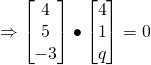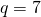(ii)
To be a parallelogram,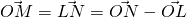(iii)
LetSince— (1)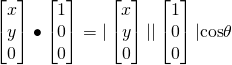— (2)
Solving,Vectors Q8 [Homework] (i)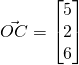(ii)
Let R be the top of the vertical pillar,Since R is collinear with A and C, R is the intersection of line AC and QR., and the height is 9m.

(iii)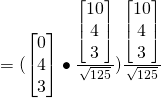Vectors Q9 [Homework] (i)Normal of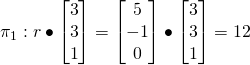(ii)
Letbe the acute angle(iii)— (1)— (2)

Using GC,(iv)
Let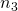be the normal ofLength of projection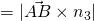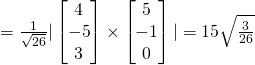(v)
Required distance(vi)
Let normal ofIfintersect at l,nlies onComments
pingbacks / trackbacks
• […] Math Sat 130pm H2 Math Sat 330pm H2 Math Sun 930am H2 Math Sun 1130am H2 Math Sun 2pm H2 Math Mon 2pm H2 Math Mon 430pm H2 Math Mon 730pm H2 Math Tue 5pm H2 Math Tue 7pm H2 Math Thur […]

Contact Us

CONTACT US We would love to hear from you. Contact us, or simply hit our personal page for more contact information

Not readable? Change text.Start typing and press Enter to search